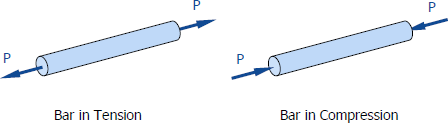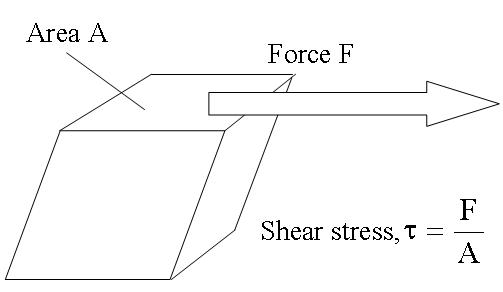# Normal and Shear Stresses

## Normal Stress

Stress is defined as the strength of a material per unit area or unit strength. It is the force on a member divided by area, which carries the force, formerly express in psi, now in N/mm² or MPa.

σ=P/A

where P is the applied normal load in Newton and A is the area in mm2. The maximum stress in tension or compression occurs over a section normal to the load.
Normal stress is either tensile stress or compressive stress. Members subject to pure tension (or tensile force) is under tensile stress, while compression members (members subject to compressive force) are under compressive stress.Compressive force will tend to shorten the member. Tension force on the other hand will tend to lengthen the member.

## Shear Stresses

A shear stress denoted$\tau\,$ (Greek: tau) is defined as the component of stress co-planar with a material cross section. Shear stress arises from the force vector component parallel to the cross section. Normal stress, on the other hand, arises from the force vector component perpendicular to the material cross section on which it acts.

Forces parallel to the area resisting the force cause shearing stress. It differs to tensile and compressive stresses, which are caused by forces perpendicular to the area on which they act. Shearing stress is also known as tangential stress.τ=F/A

where F is the resultant shearing force which passes through the centroid of the area A being sheared.

### Feedback is important to us.#### Shiva

I love to write technical articles.

error: Content is protected !!